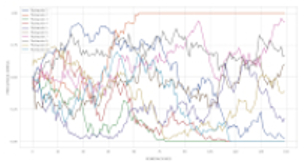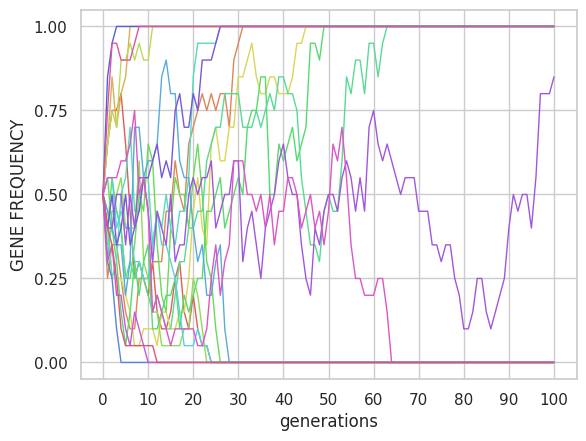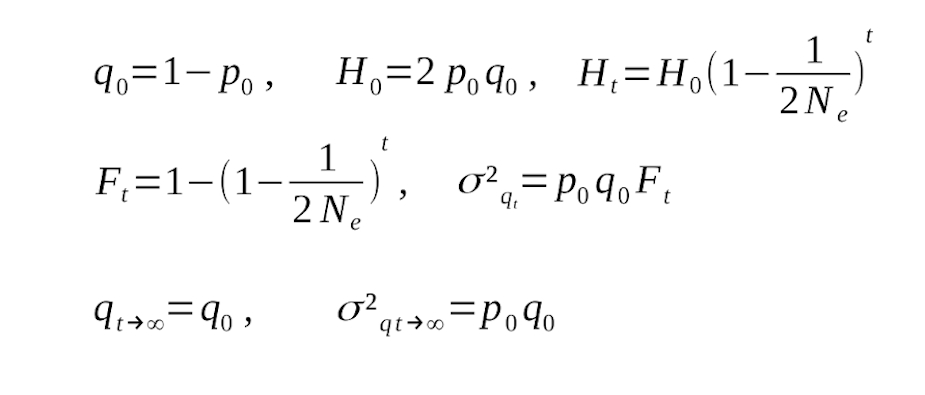﻿ 1LocusSim Manual and Exercises
1LocusSim: SIMULATION OF A LOCUS WITH GENETIC DRIFT, MUTATION AND SELECTIONFeatures
1. 1LocusSim is a simple and adaptable (mobile-friendly) simulator to visualize the effect of genetic drift, selection and mutation on allele frequency.
2. It is programmed in Python based on the NumPy library.

Contact

Back to AC-R home
Simulation of genetic drift
Genetic drift
In the simulator, the population size N corresponds to the effective population size Ne as long as there is no mutation or selection, since mating is random and by default self-fertilization is allowed. Random fluctuations in the allele frequency q will manifest as an increase in the variance of the allele frequency σ2q between different subpopulations or replicates. For the same number of populations and generations we will see that the variance increases more rapidly if Ne is low. For example, with 20 populations we can see in Figure 1 the comparison between the case Ne=10 and Ne=1000 after 100 generations of evolution.Figure 1. Effect of drift on genetic diversity. Parameters: μ=0, s=0.0. The mean frequency value in Figure 1 after 100 generations is in both cases around 0.5 but the variance with Ne=10 is close to the maximum (0.25 ) while with Ne=1000 it is 0.01. The drift generates dispersion of the frequencies in the different lines. What we observe in Figure 1 is that this dispersion occurs faster when Ne is smaller, which is visually appreciated by the separation between the different lines. This is consistent with what is predicted by the formulas for Ft and σ2q of Figure 2.Figure 2. Equations of the effect of drift on genetic diversity. The drift effect is mediated by the quantity 2Ne which, in turn, affects the variance σ2 q of the frequency between lines. In the absence of other forces, the expected allele frequency does not change but the between-line variance increases, and after a sufficient number of generations it will reach its maximum value p0q0, which occurs when all populations have their frequency at 0 or 1, that is, all lines are fixed (Ft=1). If Ne is small, this value will be reached more quickly, as we can see in Figure 1. On the contrary, if N e is large, there is less drift, and the variance increases more slowly. To appreciate the effect of the drift on the variance of the allele frequency we can use the simulator, for example, setting the number of populations to 20, playing with different population sizes and leaving the rest of the default parameters.
Exercises
Figure 2 shows the mathematical relationship between drift and genetic variability. Use the formulas that appear there to solve the following exercises.

Exercise 1
In the drift model, the variance between lines is initially 0 because the initial frequency q0 is the same for all populations. In several experiments during 3 generations the variance increased by more than 20%. The variance in the third generation was 0.21. If the initial allele frequency was 0.5 and there is no mutation or selection, what was the effective populaton size? Check it by simulation.

Initially q0=0.5 and at t=3, σ2q3=0.21 then 0.21=0.25×F3 and solving, F3=0.84. The relationship with the effective population size is 0.84=1-(1-1/(2Ne))3. Therefore (1-1/(2Ne))3=0.16. Taking the cube root of both sides of the equality and solving for Ne we get 1-1/(2Ne )=0.543 => 0.457=1/(2Ne) => Ne≈1.

Exercise 2
100 populations with initial frequency q0=0.5 were simulated. After 5 generations the variance of q was approximately 0.1. There is no mutation or selection. Would you say that the effective population size is around 5 or 50? Justify your answer and check it by means of a simulation.

Remember that the lower the effective population size, the faster the increase in variance. We know that σ2q5=p0q0F5. Since p0=q0=0.5 and σ2q5≈0.1 we have 0.1= 0.25×F5 and solving, F5=0.4.
If it were Ne=5 then F5=1-(1-1/(2Ne))5=1-(1-1/(10))5=0.41.
If it were Ne=50 then F5=1-(1-1/(2Ne))5=1-(1-1/(100))5=0.05.
With which it seems that the effective size is around 5 and not 50. Which we can easily check by performing a simulation with parameters n=100, q0=0.5, t=5 without mutation or selection and testing first Ne=5 and then Ne=50. And checking in each case the value of the variance obtained (we expect it to be approximately 0.1).

Exercise 3
What will be the minimum number of generations necessary so that in a set of populations of size 5, the variance between lines obtained is half the maximum variance? HINT: Remember that the maximum variance is p0q0.

If Ne=5 then Ft=1-(1-1/10)t=1-0.9t. Since σ2qt=p0q0Ft we have that p0q0/2=p0q0Ft and therefore Ft=0.5=1-0.9t=>0.9t=0.5. Taking logarithms to both sides, t=log(0.5)/log(0.9)=6.6≈7 will be the minimum number of generations needed.

A. Carvajal-Rodriguez - Departamento de Bioquímica Genética e Inmunología - Universidad de Vigo. ( Updated: March 2023)